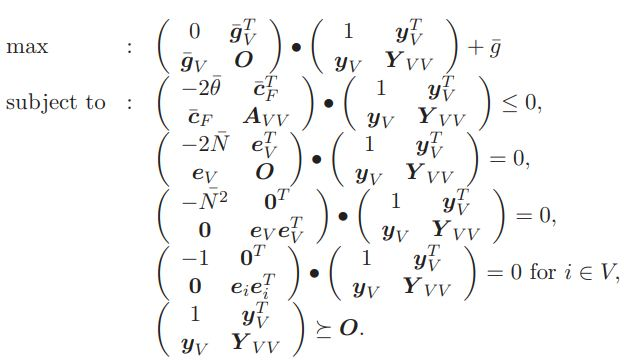# How can I eliminate Inaccurate/solved status?

How can I eliminate Inaccurate/solved status?

My original SDP problem is as followingI build the following model to solve the above SDP problem.

the sizes of array gbar_V, cbar_F, e_V are all equal to [count1] ;
thetabar, Nbar are real number.
the sizes of matrix A_VV, e_V
e_V’ are all equal to [count*count] .

%======================================
n = 1+count;
M = [0, gbar_V’; gbar_V, zeros(count)];
R1 = [-2thetabar, cbar_F’; cbar_F, A_VV];
R2 = [-2
Nbar, e_V’; e_V, zeros(count)];
R3 = [-NbarNbar, zeros(count,1)’; zeros(count,1), e_Ve_V’];

cvx_begin sdp
variable Y(n,n) semidefinite
maximize(sum(dot(M,Y))+gbar)
subject to
diag(Y) == 1
sum(dot(R1,Y)) <= 0
sum(dot(R2,Y)) == 0
sum(dot(R3,Y)) == 0
cvx_end
%======================================

when the count is small (count = 151),
the Status showed “Solved” ;
But when the count is increasing to 1001 (count = 1001),
the Status showed “Inaccurate/solved” ;
How can I eliminate Inaccurate/solved status?